## 过火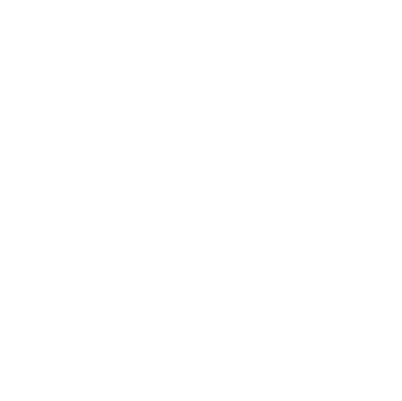1.相机补位式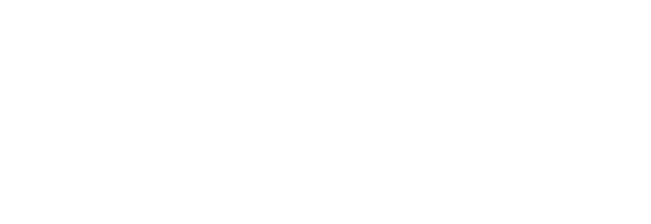（ gif 取自 https://www.gameres.com/840857.html

2.纹理采样式

```PShader shader ;
int increW;
int increH;
int WCOUNT = 10;
int HCOUNT = 10;
void drawRect(PGraphics pg, int c, int x, int y, int w, int h) {
pg.noStroke();
pg.fill(c);
pg.rect(x, y, w, h);
}
PGraphics drawOneGraphics()
{
PGraphics pg = createGraphics(width, height);
pg.beginDraw();
int k = 0;
int c = 0;
for (int x = 0; x  < width; x += increW)
{
for (int y = 0; y < height; y += increH)
{
if (k % 2 == 0)
c = color(255);
else
c = color(0);
drawRect(pg, c, x, y, increW, increH);
k++;
}
k++;
}
//pg.stroke(255,0,0);
//pg.strokeWeight(10);
//pg.noFill();
//pg.rect(0,0,width,height);
pg.endDraw();
return pg;
}
void settings() {
size(400, 400,P2D);
}
void setup() {
textureWrap(REPEAT);
increW = width/WCOUNT;
increH = height/HCOUNT;
}
void draw() {
PImage chessboard = drawOneGraphics();
image(chessboard, 0,0);
}```

，不满足需求，因此要修改为 `P2D`
,在 `size()`

。其次是将着色器 `PShader`

`shader()`

`shader()`

```image(mygraphics1,0,0,);
image(mygraphics2,0,0,);

```shader(myshader);
image(mygraphics1,0,0,);
drawMyGeometry();         //注意是放在绘制模型之前调用，作为材质着色器使用```

```#ifdef GL_ES                    //这一部分是基于平台的数值精度定义，方便优化
precision mediump float;
precision mediump int;
#endif
uniform sampler2D texture;          //等待pde中的默认graphics传入，Processing底层封装好的变量名，不能更改，如果更改名字就意味用户自己的贴图变量
uniform float time;                 //等待被传入的时间变量
uniform vec2 resolution;            //视口大小
varying vec4 vertColor;
varying vec4 vertTexCoord;
void main(void) {
vec2 p = vec2(time*0.1,time*0.1) + gl_FragCoord.xy / resolution.xy ; //待采样的目标纹理坐标
vec3 col = texture2D(texture,  p).xyz;//纹理采样
vec4 cc = vec4( col, 1.0) ;
gl_FragColor = cc;                    //输出片元颜色
}```

。要提的是 `texture2D()`

，那如何知道哪个纹理上的值是对应屏幕上的哪个点呢，使用 `gl_FragCoord.xy / resolution.xy`

，UV是标准的[0,1]相对坐标值。如果要偏移纹理图像，那幺就得在 `texture2D()`

## 回火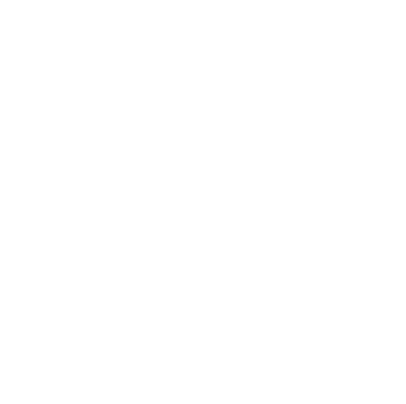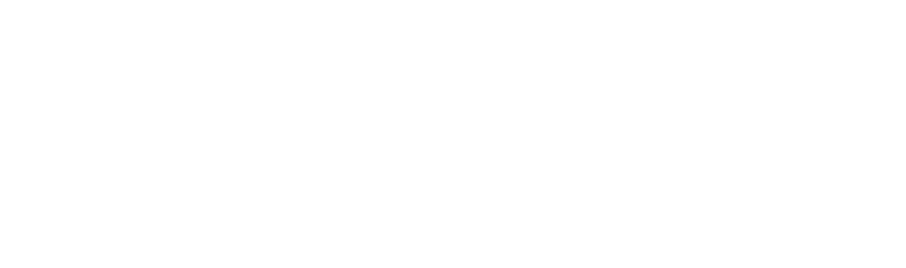400，那幺一轴向上的总像素量为 `X = 3*x + 2*y + 0.5*y*2`
.经计算，得到 `400 = 3*x + 3*y`
，其中x就是 `increX`
，那幺就很容易得出 空隙 `y = 视窗宽度 / 方块个数 - 步长increment`
。化成代码如下：

```int edageweight = 10;
int WCOUNT = 8;
int HCOUNT = 8;
int increW;
int increH;
int k = 0;
int i, j;
i = -(increW+edageweight);        //变量i辅助计算绘制起始点
j = -(increH+edageweight);        //变量j辅助计算绘制起始点
for (int x=0; x < 10; x++) {
i += increW+edageweight;
for (int y=0; y < 10; y++) {
j += increH+edageweight;
if (k % 2 == 0)
{
int c = color(200, 20, 20);
drawRect(c, i+edageweight/2, j+edageweight/2, increW, increH);
}
k++;
}
j = -(increH+edageweight);
k++;
}
i = -(increW+edageweight);```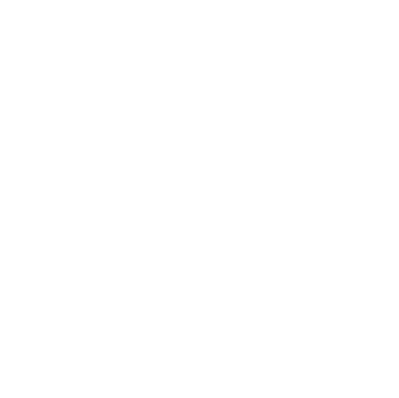pde代码：

```int WCOUNT = 8;
int HCOUNT = 8;
int increW;
int increH;
int edageweight = 10;
void settings() {
size(400,400, P2D);
}
void setup() {
textureWrap(REPEAT);
increW = (width)/WCOUNT-edageweight;
increH = (height)/HCOUNT-edageweight;
}
void draw() {
background(230);
Process();
}
void drawRect(int c, int x, int y, int w, int h) {
noStroke();
fill(c);
rect(x, y, w, h);
}
void Process()
{
int k = 0;
int i, j;
i = -(increW+edageweight);
j = -(increH+edageweight);
for (int x=0; x < 10; x++) {
i += increW+edageweight;
for (int y=0; y < 10; y++) {
j += increH+edageweight;
if (k % 2 == 0)
{
int c = color(200, 20, 20);
drawRect(c, i+edageweight/2, j+edageweight/2, increW, increH);
}
k++;
}
j = -(increH+edageweight);
k++;
}
i = -(increW+edageweight);
}```

```#ifdef GL_ES
precision mediump float;
precision mediump int;
#endif
uniform sampler2D texture;
uniform float time;
uniform vec2 resolution;
uniform vec2 mouse;
varying vec4 vertColor;
varying vec4 vertTexCoord;
void main(void) {
vec2 p =  vec2(1,-1)*mouse.xy/resolution.xy  + gl_FragCoord.xy / resolution.xy ;
vec3 col = texture2D(texture,  p).xyz;
vec4 cc = vec4( col, 1.0) ;
gl_FragColor = cc;
}```

pde中加入：

`shader.set("mouse", (float)mouseX, (float)mouseY);`